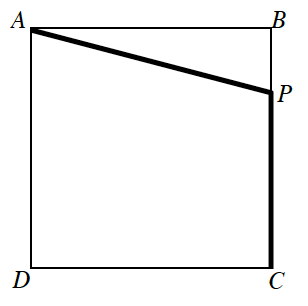### Home > APCALC > Chapter 9 > Lesson 9.1.2 > Problem9-25

9-25.

A square plot of land $100$ feet on each side is shown in the diagram at right. A pipe is to be laid in a straight line from point $A$ to a point $P$ on side $\overline{BC}$, and from there to point $C$. (Point $P$ cannot be point $B$ or point $C$.) The cost of laying the pipe is $20$ per foot if the pipe goes through the lot from point $A$ to point $P$ (since it must be laid underground) and $10$ per foot if it is laid along one of the sides of the lot. What is the most economical way to lay the pipe?Let $x = \overline {BP}$.
Now write expressions for $\overline{AB}$ and $\overline{CP}$.

The function for the cost is $y = 20(\overline{AP}) + 10(\overline{CP})$.

Once you have written the cost function in terms of $x$, differentiate and determine the value of $x$ that yields a miniumum.

$x ≈ 57.735$ ft